In this page we will explain the topics for the chapter 3 of Understanding Quadrilaterals Class 8 Maths.We have given quality notes and video to explain various things so that students can benefits from it and learn maths in a fun and easy manner, Hope you like them and do not forget to like , social share and comment at the end of the page.

## What is Closed curve and Open curve

Closed curve is a figure in the plane with no end points. It completely encloses an area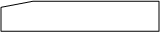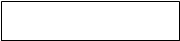Open curve is a figure in the place with end points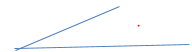## What are polygons

A simple closed curve made up of only line segments is called a polygon.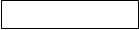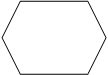## Classification of polygons

We classify polygons according to the number of sides( or vertices)
 Number of sides Classification 3 Triangle 4 Quadrilateral 5 Pentagon 6 Hexagon 7 Heptagon 8 Octagon 9 Nonagon

## Diagonal in Polygons

A diagonal is a line segment connecting two non-consecutive vertices of a polygon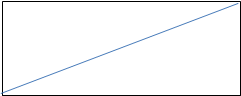## Convex and Concave Polygons

Convex Polygon
We have all the diagonals inside the Polygon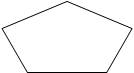Concave Polygon
We don’t have all the diagonals inside the Polygon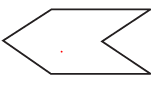We will be concentrating on Convex Polygon in this chapter

## Regular and Irregular Polygons

A regular polygon is both ‘equiangular’ and ‘equilateral’.
So all the sides and angles should be same
a) So square is a regular polygon but rectangle is not
b) Equilateral triangle is a regular polygon

## Angle Sum in the Polygons

The Sum of the angles in the polygon is given by
=(n-2) × 1800
For Triangle, n=3
So Total =1800
So total =3600

Example
Find the value of angle x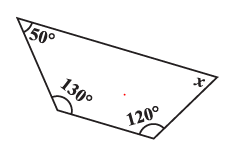Solution
We know in the quadrilateral, sum of interior angle is 3600
So
50+130+120+x=360
x=600
Watch this tutorial on how to solve angle problems in quadrilateral

## Sum of the Measures of the Exterior Angles of a Polygon

The sum of the measures of the external angles of any polygon is 360°.
This property is very useful is finding number of sides of the polygons

Example:
Find the number of sides of a regular polygon whose each exterior angle
has a measure of 60°.
Solution: Total measure of all exterior angles = 360°
Measure of each exterior angle = 60°
Therefore, the number of exterior angles =360/60=6
The polygon has 6 sides.
Watch this tutorial on how to solve exterior angles problems

A quadrilateral is a four sides Polygon. It has four angles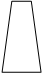Angle Sum Property
Sum of angles of the Quadrilaterals =3600
Exterior Angle Property
Sum of exterior angles of the Quadrilaterals =3600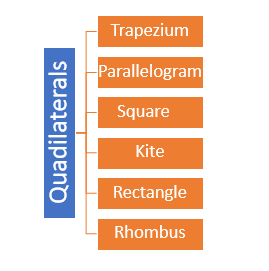### Trapezium

Trapezium is a quadrilateral with a pair of parallel sides.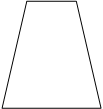### Isosceles trapezium

Trapezium when non-parallel sides of it are of equal length

### Kite

It is a quadrilaterals having exactly two distinct consecutive pairs of sides of equal length
Here ABCD is a Kite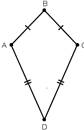AB=BC

### Parallelogram

It is a quadrilateral whose opposite sides are parallel.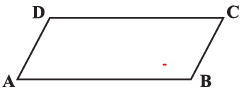Here ABCD is a Parallelogram
Property 1
The opposite sides of a parallelogram are of equal length.
Property 2
The opposite angles of a parallelogram are of equal measure
∠A= ∠C , ∠B= ∠D
Property 3
The adjacent angles in a parallelogram are supplementary.
∠A+ ∠D=180 , ∠B+ ∠C=180
Property 4
The diagonals of a parallelogram bisect each other

Example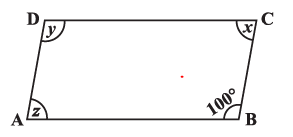Find the value of the angles x,y,z in the parallelograms given above
Solution
Angle B and Angle D are opposite angle and are equal
So y=1000
Angle C and Angle B are adjacent angle and are supplementary
So x+100=180
x=800
Angle A and Angle C are opposite angle and are equal
So z=800

### Rhombus

A rhombus is a quadrilateral with sides of equal length
A rhombus has all the properties of a parallelogram and also that of a kite.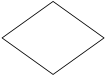Special Property
The diagonals of a rhombus are perpendicular bisectors of one another

### Rectangle

A rectangle is a parallelogram with equal angles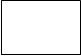Property 1
Each angle is of 900
Property 2
The diagonals of a rectangle are of equal length

### Square

A square is a rectangle with equal sides.
So it has the same property of Rectangle in addition of 4 equal sides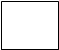Property
The diagonals of a square are perpendicular bisectors of each other.

Watch this tutorial for understanding quadrilaterals

Reference Books for class 8 Math

Given below are the links of some of the reference books for class 8 Math.

1. Mathematics Foundation Course for JEE/Olympiad : Class 8 This book can take students maths skills further. Only buy if child is interested in Olympiad/JEE foundation courses.
2. Mathematics for Class 8 by R S Aggarwal Detailed Mathematics book to clear basics and concepts. I would say it is a must have book for class 8 student.
3. Pearson Foundation Series (IIT -JEE / NEET) Physics, Chemistry, Maths & Biology for Class 8 (Main Books) | PCMB Combo : These set of books could help your child if he aims to get extra knowledge of science and maths. These would be helpful if child wants to prepare for competitive exams like JEE/NEET. Only buy if you can provide help to the child while studying.
4. Reasoning Olympiad Workbook - Class 8 :- Reasoning helps sharpen the mind of child. I would recommend students practicing reasoning even though they are not appearing for Olympiad.

You can use above books for extra knowledge and practicing different questions.

Note to our visitors :-

Thanks for visiting our website. From feedback of our visitors we came to know that sometimes you are not able to see the answers given under "Answers" tab below questions. This might happen sometimes as we use javascript there. So you can view answers where they are available by reloding the page and letting it reload properly by waiting few more seconds before clicking the button.
We really do hope that this resolve the issue. If you still hare facing problems then feel free to contact us using feedback button or contact us directly by sending is an email at [email protected]
We are aware that our users want answers to all the questions in the website. Since ours is more or less a one man army we are working towards providing answers to questions available at our website.